P-V plots for two gases during adiabatic process are shown in the figure. Plots 1 and 2 should correspond respectively to -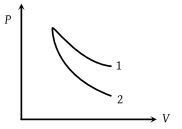(1) He and O2

(2) O2 and He

(3) He and Ar

(4) O2 and N2

Concept Questions :-

Types of processes
High Yielding Test Series + Question Bank - NEET 2020

Difficulty Level:

Four curves A, B, C and D are drawn in the adjoining figure for a given amount of gas. The curves which represent adiabatic and isothermal changes are -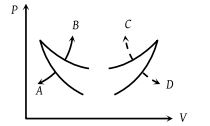(1) C and D respectively

(2) D and C respectively

(3) A and B respectively

(4) B and A respectively

Concept Questions :-

Types of processes
High Yielding Test Series + Question Bank - NEET 2020

Difficulty Level:

In pressure-volume diagram given below, the isochoric, isothermal, and isobaric parts respectively are -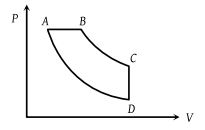(2) DC, CB, DA

(3) AB, BC, CD

(4) CD, DA, AB

Concept Questions :-

Types of processes
High Yielding Test Series + Question Bank - NEET 2020

Difficulty Level:

An ideal gas is taken through the cycle ABCA, as shown in the figure. If the net heat supplied to the gas in the cycle is 5 J, the work done by the gas in the process CA is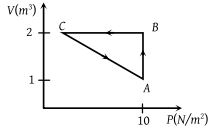(1) – 5 J

(2) – 10 J

(3) – 15 J

(4) – 20 J

Concept Questions :-

Cyclic process
High Yielding Test Series + Question Bank - NEET 2020

Difficulty Level:

In the following indicator diagram, the net amount of work done will be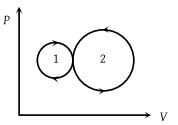(1) Positive

(2) Negative

(3) Zero

(4) Infinity

High Yielding Test Series + Question Bank - NEET 2020

Difficulty Level:

A cyclic process for 1 mole of an ideal gas is shown in figure in the V-T, diagram. The work done in AB, BC and CA respectively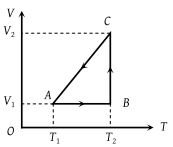(1) $0,\text{\hspace{0.17em}}R{T}_{2}\mathrm{ln}\left(\frac{{V}_{1}}{{V}_{2}}\right)\text{\hspace{0.17em}},\text{\hspace{0.17em}}R\text{\hspace{0.17em}}\left({T}_{1}-{T}_{2}\right)$

(2) $R\left({T}_{1}-{T}_{2}\right),\text{\hspace{0.17em}}0,\text{\hspace{0.17em}}R{T}_{1}\mathrm{ln}\frac{{V}_{1}}{{V}_{2}}$

(3) $0,\text{\hspace{0.17em}}R{T}_{2}\mathrm{ln}\left(\frac{{V}_{2}}{{V}_{1}}\right)\text{\hspace{0.17em}},\text{\hspace{0.17em}}R\text{\hspace{0.17em}}\left({T}_{1}-{T}_{2}\right)$

(4) $0,\text{\hspace{0.17em}}R{T}_{2}\mathrm{ln}\left(\frac{{V}_{2}}{{V}_{1}}\right)\text{\hspace{0.17em}},\text{\hspace{0.17em}}R\text{\hspace{0.17em}}\left({T}_{2}-{T}_{1}\right)$

High Yielding Test Series + Question Bank - NEET 2020

Difficulty Level:

A cyclic process ABCD is shown in the P-V diagram. Which of the following P-T curves represent the same process ?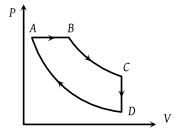(1)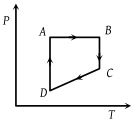(2)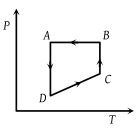(3)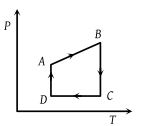(4)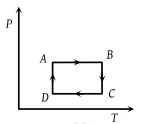Concept Questions :-

Types of processes
High Yielding Test Series + Question Bank - NEET 2020

Difficulty Level:

Carnot cycle (reversible) of a gas represented by a Pressure-Volume curve is shown in the diagram . Consider the following statements

I. Area ABCD = Work done on the gas

II. Area ABCD = Net heat absorbed

III. Change in the internal energy in cycle = 0

Which of these are correct ?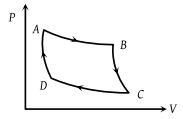(1) I only

(2) II only

(3) II and III

(4) I, II and III

Concept Questions :-

First law of thermodynamics
High Yielding Test Series + Question Bank - NEET 2020

Difficulty Level:

The temperature-entropy diagram of a reversible engine cycle is given in the figure. Its efficiency is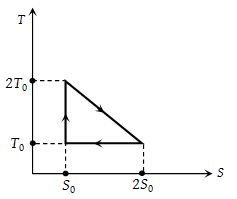(1) 1/3

(2) 2/3

(3) 1/2

(4) 1/4

Concept Questions :-

Heat engine and refrigerator
High Yielding Test Series + Question Bank - NEET 2020

Difficulty Level:

Work done in the given P-V diagram in the cyclic process is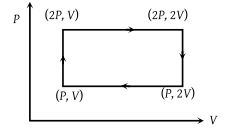(1) PV

(2) 2PV

(3) PV/2

(4) 3PV

Concept Questions :-

Work done by gas